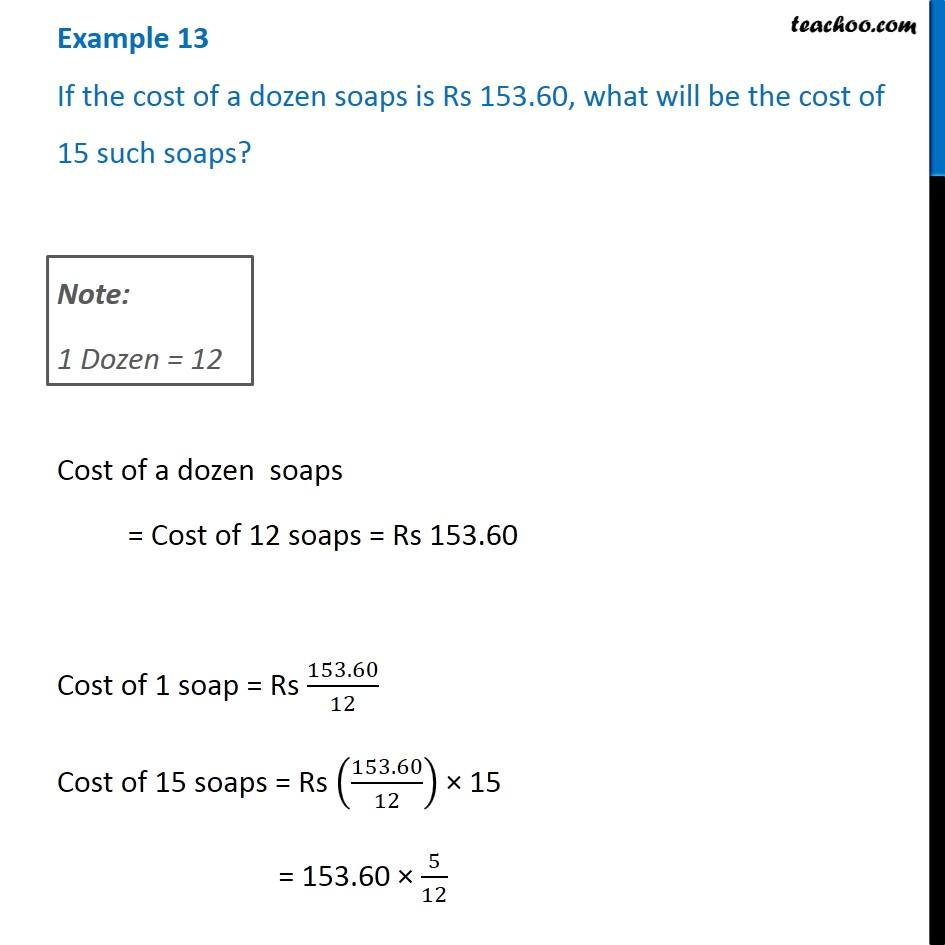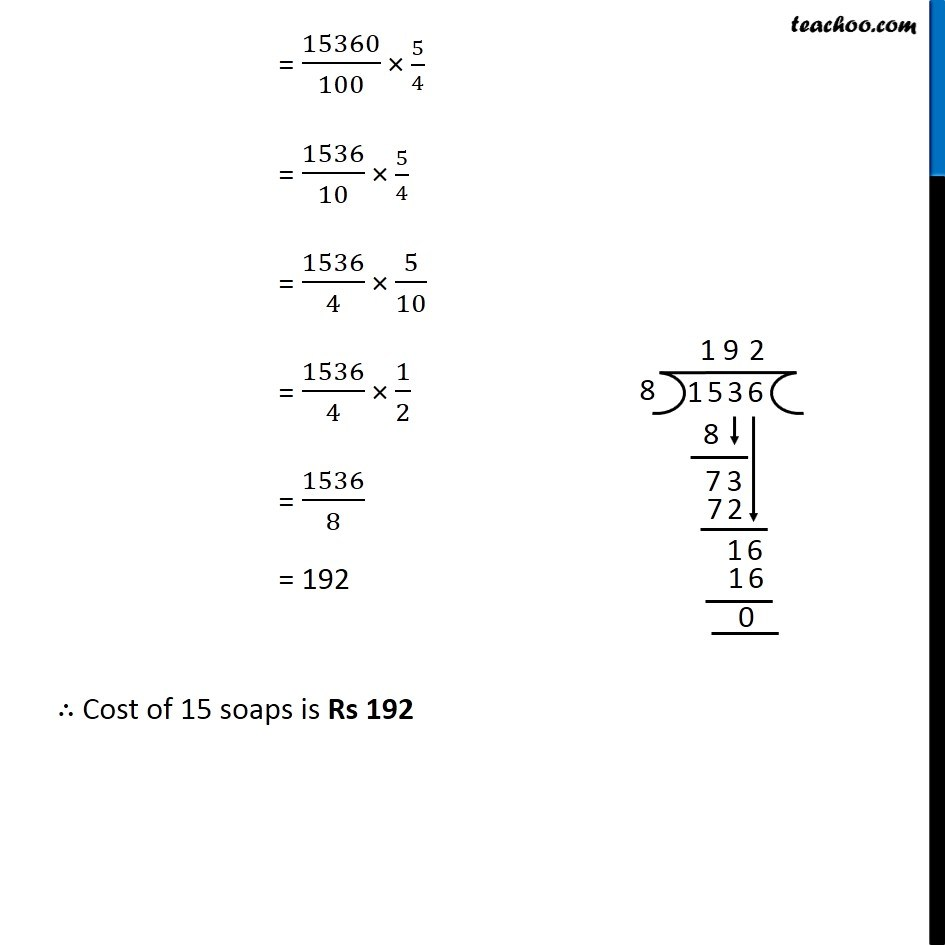Unitary Method

Chapter 12 Class 6 Ratio And Proportion
Concept wiseIntroducing your new favourite teacher - Teachoo Black, at only ₹83 per month

### Transcript

Example 13 If the cost of a dozen soaps is Rs 153.60, what will be the cost of 15 such soaps? Note: 1 Dozen = 12 Cost of a dozen soaps = Cost of 12 soaps = Rs 153.60 Cost of 1 soap = Rs 153.60/12 Cost of 15 soaps = Rs (153.60/12) × 15 = 153.60 × 5/12 = 15360/100 × 5/4 = 1536/10 × 5/4 = 1536/4 × 5/10 = 1536/4 × 1/2 = 1536/8 = 192 ∴ Cost of 15 soaps is Rs 192